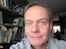# Math Is Fun Forum

Discussion about math, puzzles, games and fun.   Useful symbols: ÷ × ½ √ ∞ ≠ ≤ ≥ ≈ ⇒ ± ∈ Δ θ ∴ ∑ ∫  π  -¹ ² ³ °

You are not logged in.

## #1 2023-08-18 01:02:45

Ivar Sand
MemberRegistered: 2013-01-22
Posts: 16

### 2 + 2 = 4

We intend to prove that 2 + 2 = 4.

We start by defining the sequence of natural numbers (1):
1, 2, 3, ... s,t, ...
Here, s, and t represent two consecutive natural numbers.

So far in our presentation, the natural numbers are only symbols.
We define their values by introducing the value equation, which defines uniquely the value of every natural number except for 1 in terms of the value of 1:
for every natural number s: t = s + 1
The natural number s should go through the natural numbers sequentially from 1 so that the right-hand side of the value equation is defined for every value of s.

To make the definition of the value equation complete, we should define the +-operator.
However, we choose instead to introduce an axiom of the natural numbers, the associativity axiom (2):
for every natural number a, b, and c: a + (b + c) = (a + b) + c

We are now in a position to prove:

Theorem:
2 + 2 = 4
Proof:
We operate on the left-hand side of the equation 2 + 2 = 4:
2 + 2
= 2 + (2)         as a parenthesis can be put around a singular natural number
= 2 + (1 + 1)   as 2 = 1 + 1 by the value equation
= (2 + 1) + 1   by the associativity axiom
= (3) + 1         as 3 = 2 + 1 by the value equation
= 3 + 1           as a parenthesis around a singular natural number can be removed
= 4                 as 4 = 3 + 1 by the value equation
We see from this that we have proved that 2 + 2 = 4.
This is what we intended to prove, so the proof is complete.

References:
1 Natural number https://en.wikipedia.org/wiki/Natural_number
2 Associative Laws https://www.mathsisfun.com/associative-commutative-distributive.html

Last edited by Ivar Sand (2023-08-22 01:09:45)

I majored in Physics in 1976. Also, I studied mathematics and computer science. I work as a computer programmer. I am from Norway.

Offline

## #2 2023-08-18 01:53:04

Jai GaneshRegistered: 2005-06-28
Posts: 43,558

### Re: 2 + 2 = 4

Hi Ivar Sand,

A good post.

Incidentally, there are topics in Natural Number and Associative Property in this context.

It appears to me that if one wants to make progress in mathematics, one should study the masters and not the pupils. - Niels Henrik Abel.

Nothing is better than reading and gaining more and more knowledge - Stephen William Hawking.

Offline